\$1,850.00

# fx-CG50

## Easy-to-operate, high-definition 3D Graph provides visual support for mathematical exploration.

fx-CG50 has intuitive operability and exploring features, ideal for mathematics learning.

OS Update Information

• Python function is updated.

# Features

## 1 Easy and Intuitive operability

Intuitive operability and G-Solve features make it easy to use the features of fx-CG50.

So you can spend less time teaching how to use graphing calculators (, and more time teaching mathematics).

•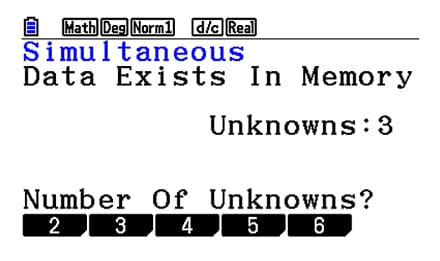Interactive format

•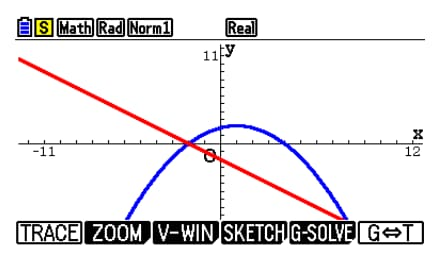G-Solve feature

## 1Intuitive operation

The user-friendly Icon menu, Function keys and Interactive format enable intuitive operation.

•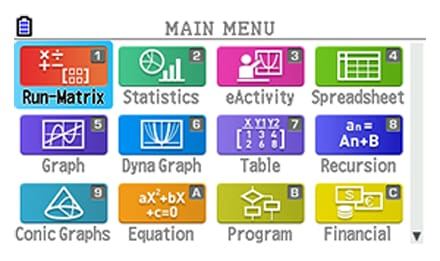Icon menu

••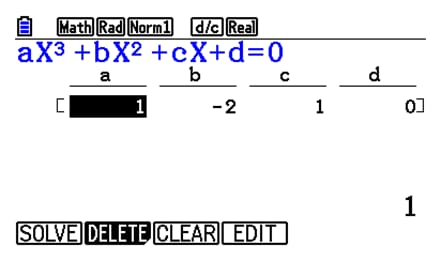Function keys

••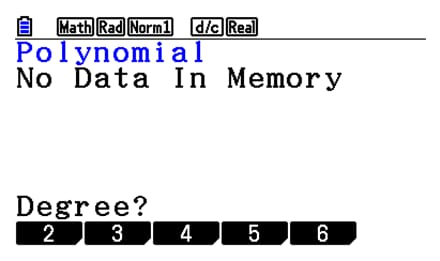Interactive format

## Example

• Solve the Polynomial equation.
x2 + 2x + 1 = 0
• The solution is
x = -1
•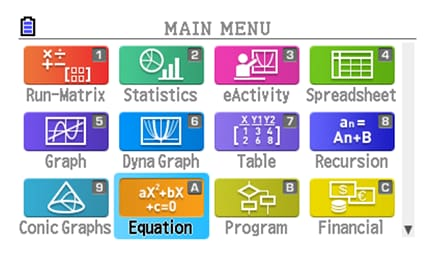Icon display

••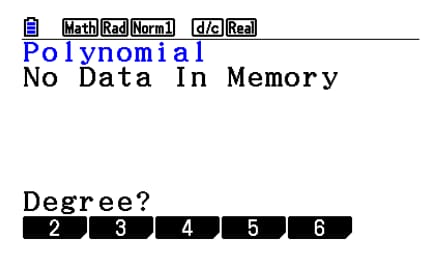Interactive format

••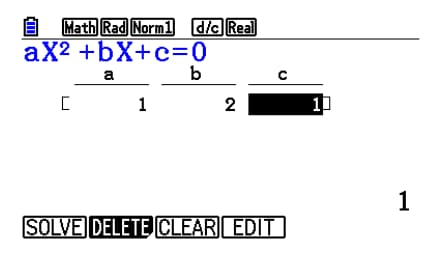Input coefficient

••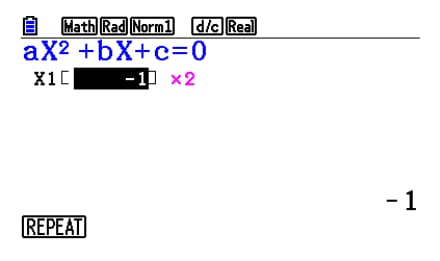Solution

## 2G-Solve feature

Use the G-Solve feature to easily solve problems involving intersections, roots, and integration.

•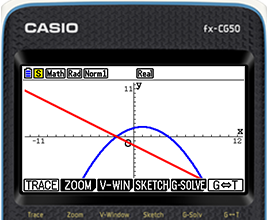•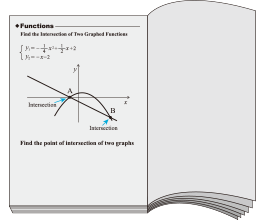Find the point of intersection of two graphs by simply pressing the “G-Solv” key.

## 2 Essential functions for learning all in one

Graphing calculators have a variety of functions covering from primary to higher education. Students can cultivate mathematical thinking through Graph functions operated by themselves.

•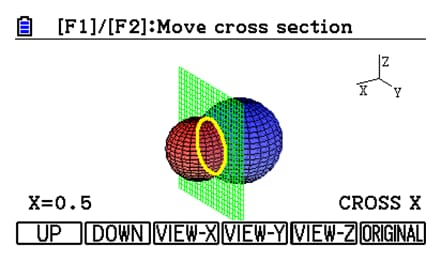3D Graph function

Email : sales@csstationery.hk

Fax : (852) 2397 5676
WhatsApp : (852) 6174 1284